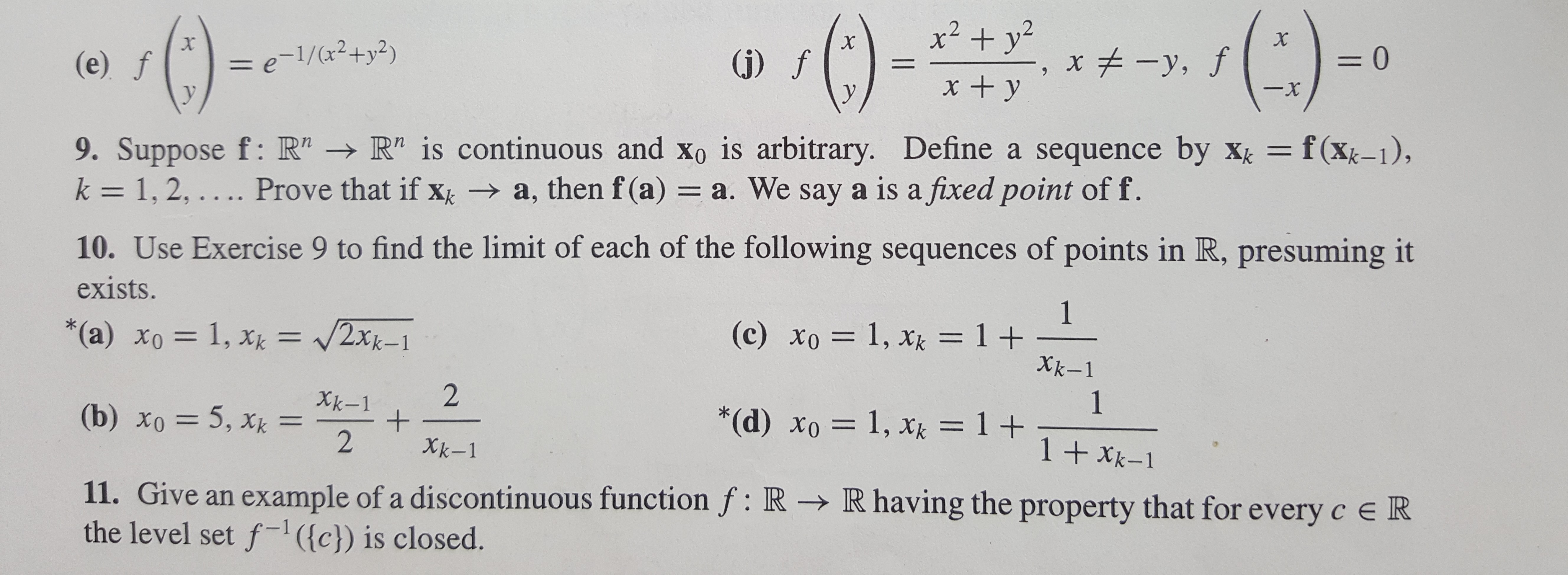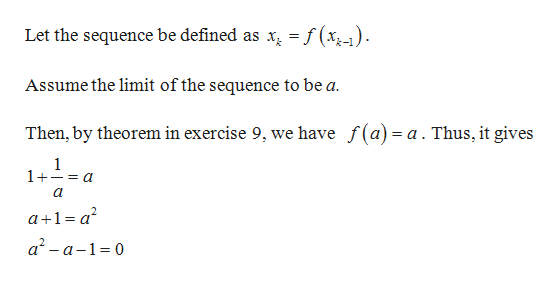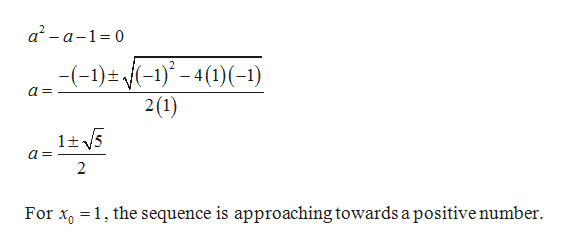x2+yx , JX= e-1/(2+y2)=0x-,f(e) f(j)fxy-xy9. Suppose f: R" R" is continuous and Xo is arbitrary. Define a sequence by Xk = 1, 2, .. .. Prove that if x a, then f (a) a. We say a is a fixed point of f.= f(xk-1),10. Use Exercise 9 to find the limit of each of the following sequences of points in R, presuming itexists.1(c) xo 1, xk = 1 +Xk-1V2Xk-1*(a) xo= 1, xk21*(d) xo 1, xk = 1 T 1 +Xk-1Xk-1+2(b) xo 5, x =1Xk-111. Give an example of a discontinuous function f: R - R having the property that for every c e Rthe level set f({c}) is closed.

Question

Problem 9 is included in this picture.

I only need help for problem 10c. Thank you very much!help_outlineImage Transcriptionclosex2+yx , J X = e-1/(2+y2) =0 x-,f (e) f (j)f xy -x y 9. Suppose f: R" R" is continuous and Xo is arbitrary. Define a sequence by X k = 1, 2, .. .. Prove that if x a, then f (a) a. We say a is a fixed point of f. = f(xk-1), 10. Use Exercise 9 to find the limit of each of the following sequences of points in R, presuming it exists. 1 (c) xo 1, xk = 1 + Xk-1 V2Xk-1 *(a) xo= 1, xk 2 1 *(d) xo 1, xk = 1 T 1 +Xk-1 Xk-1 + 2 (b) xo 5, x = 1 Xk-1 11. Give an example of a discontinuous function f: R - R having the property that for every c e R the level set f({c}) is closed. fullscreen
Step 1

Given:

Step 2

To find: The limit of the sequence.help_outlineImage TranscriptioncloseLet the sequence be defined as x = f(x,-) Assume the limit of the sequence to be a Then, by theorem in exercise 9, we have f(a)= a. Thus, it gives 1 1+- a a+1a2 a2-a-1 0 fullscreen
Step 3

Solve for a....help_outlineImage Transcriptionclosea2-a-1 0 -(-1)-1)()-1) 2(1) lt5 2 For x1, the sequence is approaching towards a positive number fullscreen

Want to see the full answer?

See Solution

Want to see this answer and more?

Our solutions are written by experts, many with advanced degrees, and available 24/7

See Solution
Tagged in

Math